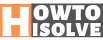# Top Math Solver Apps for iOS and Android 2020

Last Updated on Nov 10, 2020

Stuck in between the math problem? You are lucky to be born in this century because now you have hundreds of solutions to one problem. In this post, we are going to discuss that only. Math problems are good until you understand and able to solve in a minute or two. Or else there is no way out to get the answer of math problem without taking help of an expert. Therefore, we have collected a few best math solver apps for iOS and Android, that will find out the answer of math problem by scanning the problem.

It is impossible to have a math teacher whenever you want to complete your assignment or want to prepare for the test. At that time, one of the apps will help you solve the math problem. You might be thinking of how these apps solve math problems by taking pictures? It is not as simple as that, many developers and math experts have been behind the development of these apps. Help yourself with one of these apps.

Page Contents

## Best Math Solver App for iOS and Android

### #1. Photomath

Photomath is the best math solver app for iOS as well as Android devices, with the ability to solve problems based on decimals, graphs, integers, algebraic expressions, and more. Only you have to place the camera over the math problem, the smart calculator will give you result right away. Moreover, the answer given by Photomath app will be in a detailed manner, so that you can learn the problem and next time solve it by own. Even, it allows you to solve problems by writing on the iPhone or any smartphone’s screen, no need to scan and wait for the results. Photomath supports over 36 different languages for better understanding and people from anywhere learn math in their mother tongue. Here’s developer portal

Solve your Math problem instantly with intelligent PhotoMatch good for parents & better for teachers

### #2. MathwayMathwayMath Solver App

Horrified by troubling math problems? Mathway is your ultimate option to get rid of such math problems and understand them. Mathway math solver app for iPhone and Android can efficiently show you the way to solve the hardest math problem. The motto of Mathway app is to guide you with the easiest tricks to solve the math problem, not like just delivering the math answer.

If you are getting a direct answer to math problems from apps, that won’t make any progress and at the end, you might fail in exams. Therefore it is better to learn some tips and solve math problems with Mathway app.

An ideal platform to Solve the math problem on Web also. Thanks, Mathway here’s a developer website

### #3. WolframAlpha

WolframAlpha has finally gained the users and of course, it is the finest source to get quick results of computational problems. WolframAlpha app that solves a math problem by taking pictures uses a vast collection of algorithms that eventually updated by the experts to compute various math problems in a second. Following areas are covered by this app, Elementary Math, Algebra, Calculus, Number Theory, Definitions, Geometry, Matrices and much more. Not only Mathematics problems can be calculated, but also Physics, Chemistry, Life Science, Computational Science, Units and Measure, Date & Time, etc. For detailed information, you may open the following link. I would highly recommend this app.

### #4. Socratic Math

Most of the people take assistant of Socratic Math and finishes their assignments. This app takes a photo of the math problem and solves them. With the stepwise instructions, students could learn to solve the toughest math problems by heart. Besides, you can access multiple tutorials, explanations, and other material to get instant answers. It offers useful video tutorial from Khan Academy, Crash Course and other providers for better understanding.

### #5. Cymath

Got stuck with a weird math problem? Don’t worry Cymath math solver app will help you with the best techniques. Variety of topics are covered by Cymath app, so whether you want to solve Algebra or Calculus, just open the app and get started. For further assistance, you can go for Cymath Plus subscription, which will cost you less than \$5/mo and you will have personal tuition teacher at your home. Otherwise, Cymath can get you answers of maths and get your homework done in time.

### #6. MyScript Calculator

MyScript Calculator works excellent when you want to solve the problem right on your device, other than just scanning and getting answers. Do you know what is the advantage of solving math problems like that?

The questions you wrote on the app to get the answers, are remembered by you and at the time of exams, a quick strike could help you solve the problems in the correct way. Without any scratching and erasing, you can solve the math problem with MyScript Calculator app on a smartphone.

### #7. MalMath

MalMath is quick math problem app that works offline and yes it is free to download. This math solver app for Android has mastery in solving Integrals, Limits, Equations, Trigonometry, Logarithms, Derivatives, and Algebra. So if you want to solve any of the problems and have an Android phone, then go for MalMath.

The detailed description and explanation for a problem make easier to understand and increases our catch up power. MalMath offers different languages such as Portuguese, German, Turkish, Croatian, English, Russian, Japanese, French, Italian, Arabic and Azerbaijani.